## Decibels and Half Power Point Method:

Power Measurement in Decibels and Half Power Point Method – The power gain (Ap) of an amplifier may be expressed in terms of the log of the ration of output power (po) to input power (pi). This is illustrated in Fig. 8-4.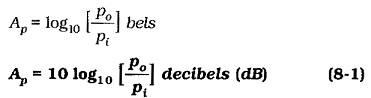Thus, the decibel is the unit of power gain, or power level change.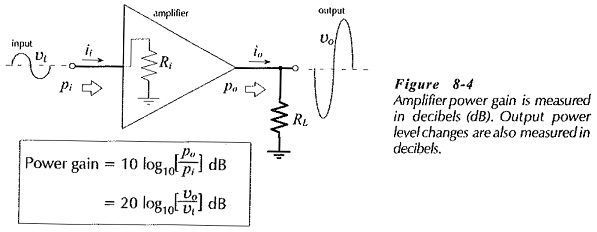The power dissipated in a resistance is (v2/R).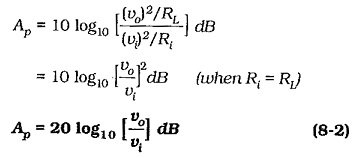Also, Po = io2 RLand Pi = i2i Ri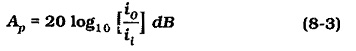It is important to note that the above equations are correct only when the load resistance connected to the circuit output is equal to the circuit input resistance. However, it is common practice in the electronics industry to apply these equations regardless of the relationship between input and load resistances!

Amplifier output power level changes (ΔP0) are also measured in decibels, and in this case the equations become,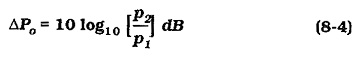where p1 is the initial output power level, and p2 is the new level.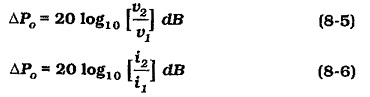where v1 and i1 are the initial output voltage and current levels, and v2 and i2 are the new levels. Equations 8-4 through 8-6 are correct so long as the amplifier load resistance remains constant.

### Half-Power Points:

Figure 8-5 shows a typical graph of amplifier output voltage or power plotted versus frequency. Note that the frequency scale is logarithmic. The output normally remains constant over a middle range of frequencies and falls off at low and high frequencies, due to effects explained in Sections 8-3 and 8-4. The gain over this middle range is termed the mid-frequency gain. The low frequency and high frequency at which the gain falls by 3 dB are designated f1 and f2, respectively. This range is normally considered the useful range of operating frequency for the amplifier, and the frequency difference (f2 – f1) is termed the amplifier bandwidth (BW).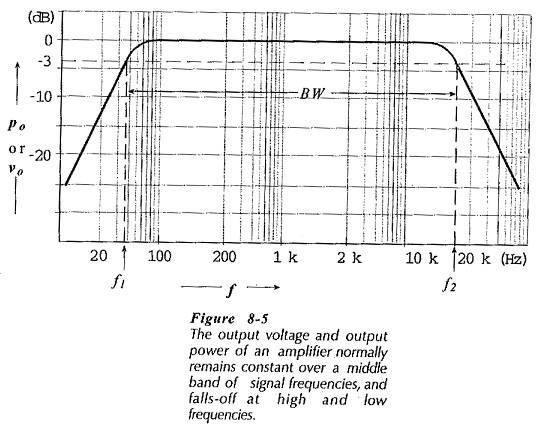Frequencies f1 and f2 are termed the half-power points, or the 3 dB points. The power output is -3 dB from its normal level when p2 is half p1. When the amplifier output is expressed as a voltage on the graph of frequency response, the 3 dB points (f1 and f2) occur when v2 is 0.707 v1.

Scroll to Top